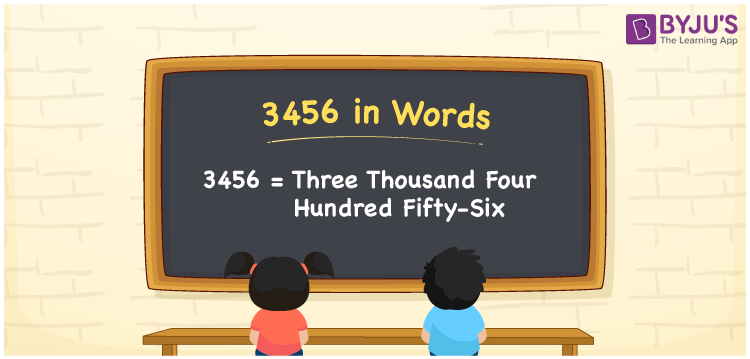# 3456 in Words

The numeral 3456 in words is “three thousand four hundred fifty-six”. The place value system plays a major role in converting numbers into words. For example, the number 3456 has four-digits, and its place values help to write the number 3456 in words. In this article, we will discuss how to spell the number 3456 in English words in detail.

 3456 in Words: Three Thousand Four Hundred Fifty-six. Three Thousand Four Hundred Fifty-six in Numerical Form: 3456.

## 3456 in English Words## How to Write 3456 in Words?

The natural number 3456 has 4 digits and its place values are given below.

 Thousands Hundreds Tens Ones 3 4 5 6

The expanded form of 3456 is as follows:

= 3 × Thousand + 4 × Hundred + 5 × Ten + 6 × One

= 3 × 1000 + 4 × 100 + 5 × 10 + 6 × 1

= 3000 + 400 + 50 + 6

= 3456

= Three thousand four hundred fifty-six

Hence, 3456 in words is three thousand four hundred fifty-six.

3456 in words – Three thousand four hundred fifty-six

Is 3456 an odd number? – No

Is 3456 an even number? – Yes

Is 3456 a perfect square number? – No

Is 3456 a perfect cube number? – No

Is 3456 a prime number? – No

Is 3456 a composite number? – Yes

## Frequently Asked Questions on 3456 in Words

Q1

### Write 3456 in words.

3456 in words is three thousand four hundred fifty-six.

Q2

### Simplify 3400 + 56, and express it in words.

Simplifying 3400 + 56, we get 3456. Hence, 3456 in words is three thousand four hundred fifty-six.

Q3

### Is 3456 a prime number?

No, 3456 is not a prime number.